Need Help?

Subscribe to Physics 1

###### \${selected_topic_name}
• Notes

$\begin{array}{c}{\text { You push your physics book } 1.50 \mathrm{m} \text { along a horizontal table- }} \\ {\text { top with a horizontal push of } 2.40 \mathrm{N} \text { while the opposing force of }} \\ {\text { friction is } 0.600 \mathrm{N} \text { . How much work does each of the following }} \\ {\text { forces do on the book: (a) your } 2.40-\mathrm{N} \text { push, }(\text { b) the friction force, }} \\ {\text { (c) the normal force from the tabletop, and (d) gravity? (e) What is }} \\ {\text { the net work done on the book? }}\end{array}$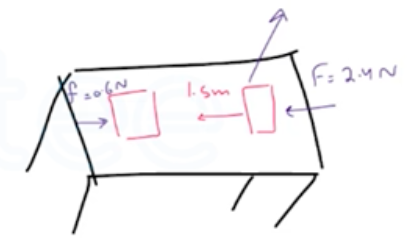$w=F s \cos \theta$

$w_{s}=(2.4) \cos 0(1.5)=3.6 \mathrm{J}$

$W_{f}=\left(F_{f} \cos \theta\right) s=(0.6 \cos 180)(1.5)$

$=-0.9 J$

$w_{n}=n \cos \theta s=n \cos 90s=0$

$W_{g r a v}=m g \cos \theta s=m g \cos 270 s=0 J$

$W_\text { net }=W_{s+} W_{f}+W_{n}+W_{g r av}=3.6-0.9+0+0=2.7 J$

$\begin{array}{c}{\text { A tow truck pulls a car } 5.00 \mathrm{km} \text { along a horizontal roadway }} \\ {\text { using a cable having a tension of } 850 \mathrm{N} \text { . ( a) How much work does }} \\ {\text { the cable do on the car if it pulls horizontally? If it pulls at } 35.0^{\circ}} \\ {\text { above the horizontal? (b) How much work does the cable do on the }} \\ {\text { tow truck in both cases of part (a)? (c) How much work does grav- }} \\ {\text { ity do on the car in part (a)? }}\end{array}$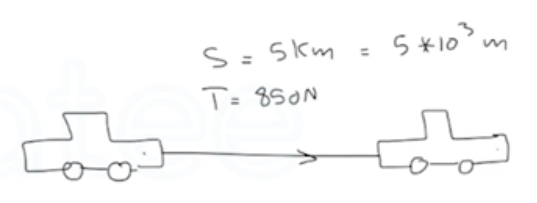$\theta=0$

$w=Fs \cos \theta$

$=850\left(5+10^{3}\right) \cos 0=4.26 \times 10^{6} \mathrm{J}$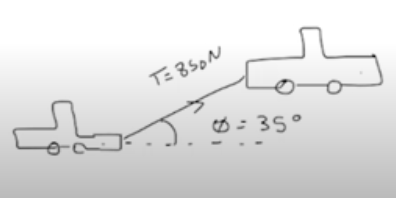$w=F s \cos \theta$

$=(850)\left(5 \times 10^{3}\right) \cos (35)=3.48+10^{6} J$

$\theta=0 \longrightarrow w \uparrow$

$\theta=\uparrow \longrightarrow w \downarrow$

$\theta=90,270 \rightarrow w=0$

$\begin{array}{l}{\text { Suppose the worker in Exercise } 6.3 \text { pushes downward at an }} \\ {\text { angle of } 30^{\circ} \text { below the horizontal. (a) What magnitude of force }} \\ {\text { must the worker apply to move the crate at constant velocity? }} \\ {\text { (b) How much work is done on the crate by this force when the }} \\ {\text { crate is pushed a distance of } 4.5 \mathrm{m} ?(\mathrm{c}) \text { How much work is done on }} \\ {\text { the crate by friction during this displacement? (d) How much work }} \\ {\text { is done on the crate by the normal force? By gravity? (e) What is }} \\ {\text { the total work done on the crate? }}\end{array}$

${F}=\quad V_ \text { constant } \rightarrow a=0 \longrightarrow \sum F=ma$

$\sum F=0$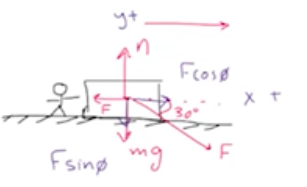$\rightarrow\sum F_{x}= F{\cos \theta}-F_{k}=0$

$F \cos \theta-F_{k}=0$

$\rightarrow \sum F_{y}=0$

$n-m g-F \sin \theta=0 \rightarrow n=m g+F \sin \theta$

$f_{k}=\mu_{k} n=\mu_{k}\left(m g+F \sin \theta\right)=\mu_{k}m g+\mu_{k} F \sin \theta$

$\sum F_{x}=F{\cos } 30-\left(\mu_{k} m g+\mu_{k} F \sin \theta\right)=0 \rightarrow F{\cos 30}- \mu_{k} mg-\mu_{k} F \sin \theta=0$

$F \cos 30-(0.25)(30)(9.81)-(0.25)(F) \sin 30=0$

$F(\cos 30-(0.25) \sin 30)-73.575=0$

$\rightarrow F(0.741)=73.575$

$∴ F=\frac{73.575}{0.741}=99.2 \mathrm{N}$

$w=F s \cos \theta \rightarrow W_{F}=(99.2)(4.5) \cos 30=387 \mathrm{J}$

$f_{k}=\mu_{k} m g+\mu_{k} F_{\sin \theta}=0.25(30)(9.8)+(0.25)(99.2) \sin 30=85.9 N$

$W_{f_k}=F_{k} S \cos \theta=85.9(4.5) \cos 180=-387 J$

$\cos 90=0 \rightarrow W_{n}=0, \quad W_{g}=0 \rightarrow \theta=270$

$W_{total}=W_{f}+W_{f_{K}}+W_{n+}W_{g}=387-387+0+0=0J$

$\begin{array}{l}{\text { A boxed } 10.0 \text { -kg computer monitor is dragged by friction }} \\ {5.50 \mathrm{m} \text { up along the moving surface of a conveyor belt inclined at }} \\ {\text { an angle of } 36.9^{\circ} \text { above the horizontal. If the monitor's speed is a }} \\ {\text { constant } 2.10 \mathrm{cm} / \mathrm{s} \text { , how much work is done on the monitor by }} \\ {\text { (a) friction, (b) gravity, and (c) the normal force of the conveyor belt? }}\end{array}$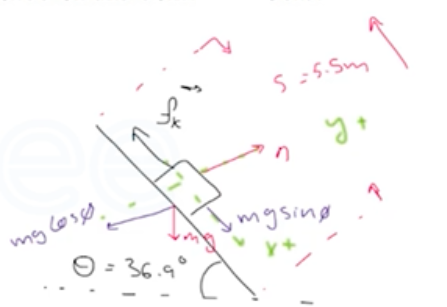$\sum F_{x}=0 \longrightarrow f_{k}-m g \sin \theta=0$

$\rightarrow f_{k}=m g \sin \theta=10(9.8) \sin 36.9$

$=58.9 N$

$w _{f_ k}=f_{k} s \cos \theta=58.9(5.5) \cos 0=324 \mathrm{J}$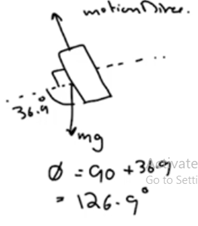$w_{g}=m g \cos \theta=10(9.81) \cos (126.9)$

$=-324 \mathrm{J}$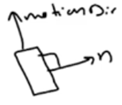$∵ \theta=90^{\circ} \rightarrow \cos 90=0$

$w_{n}=0 J$